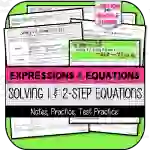Math > Algebra | Grade 7 | Activities

# Solving One- & Two-Step Equations with Rational Numbers NOTES & PRACTICEAttributes
Subject

Algebra

Types

Activities

File

PDF

Editable
NoSolving One- & Two-Step Equations with Rational Numbers NOTES & PRACTICE is a robust teaching tool specifically tailored to meet the partial requirements of the 7th Grade Expressions & Equations Standards. It's aimed at helping teachers in imparting wisdom about how variables can represent quantities in real-world or mathematical problems, and how simple equations and inequalities can be constructed.

This teaching aid focuses on CCSS.MATH.CONTENT.7.EE.B.4 and CCSS.MATH.CONTENT.7.EE.B.4.A standards, assisting educators in effectively solving word problems that include equations like px + q = r &p(x + q) = r, where rational numbers are fluently recognized as p, q, and r.

The key components of this resource include:

• 'Solving One-Step Equations with Rational Numbers NOTES & PRACTICE'
• 'Solving Two-Step Equations with Rational Numbers NOTES & PRACTICE'

This formats aids students to progressively understand these concepts while allowing their progress to be monitored by educators at each step.

In addition, it encompasses a 'TEST PRACTICE' section which comes both as printable versions and Google Forms options rendering flexibility based on the classroom needs.

A unique feature is its adaptable compatibility with various learning structures - whole group sessions for conventional classrooms; small group activities for cooperative learners or individual assignments ideal for homeschoolers/self-paced learners. It also extends into practicing stages of learning plans where students can revisit concepts without time constraints.

An answer key accompanies all segments making grading simpler by saving teachers' time and providing immediate feedback possibilities. Available in PDF format, this tool allows for easy sharing with students or printing hard copies as per the preference of educators whilst ensuring that all math content appears consistently across different platforms.

In essence, Solving One- & Two-step Equations With Rational Numbers NOTES & PRACTICE offers a flexible and stepwise approach in teaching 7th graders key algebraic concepts, adapting to both traditional classrooms and remote learning environments.

## What's Included

This resource contains the following items:

1) Solving One-Step Equations with Rational Numbers NOTES & PRACTICE

2) Solving Two-Step Equations with Rational Numbers NOTES & PRACTICE

3) Solving One- and Two-Step Equations TEST PRACTICE (Printable and version for Google Forms)

4) Answer Key to All Parts

## Resource Tags

One-step equations Two-step equations Rational numbers Expressions & Equations Word problems

### you may also like...

Check out these other great products